Difference between revisions of "Understanding mathematical definitions"

Understanding a definition in mathematics is a pretty complicated and laborious process. The following table summarizes some of the things one might do when trying to understand a new definition.

Step Condition Description Purpose Example
Type-checking and parsing
Checking assumptions of objects introduced Remove or alter each assumption of the objects that have been introduced in the definition to see why they are necessary.
Come up with examples Come up with some examples of objects that fit the definition. Emphasize edge cases. Examples help to train your intuition of what the object "looks like". For monotone increasing functions, an edge case would be the constant function.
Come up with counterexamples
Writing out a wrong version of the definition See this post by Tim Gowers (search "wrong versions" on the page).
Understand the kind of definition Generally a definition will do one of the following things: (1) it will construct a brand new type of object (e.g. definition of a function); (2) it will take an existing type of object and create a predicate to describe some subclass of that type of object (e.g. take the integers and create the predicate even); (3) it will define an operation on some class of objects (e.g. take integers and define the operation of addition).
Check that it is well-defined If the definition defines an operation
Check consistency with existing definition If the definition supersedes an older definition or it clobbers up a previously defined notation Addition on reals after addition on rationals have been defined.

For any function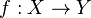$f:X\to Y$ and$U\subset Y$, the inverse image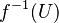$f^{-1}(U)$ is defined. On the other hand, if a function$f : X\to Y$ is a bijection, then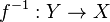$f^{-1} : Y \to X$ is a function, so its forward image$f^{-1}(U)$ is defined given any$U\subset Y$. We must check that these two are the same set (or else have some way to disambiguate which one we mean). (This example is mentioned in both Tao's Analysis I and in Munkres's Topology.)
Disambiguate similar-seeming concepts The idea is that sometimes, two different definitions "step on" the same intuitive concept that someone has. (Example from Tao) "Disjoint" and "distinct" are both terms that apply to two sets. They even sound similar. Are they the same concept? Does one imply the other? It turns out, the answer is "no" to both: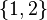$\{1,2\}$ and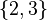$\{2,3\}$ are distinct but not disjoint, and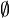$\emptyset$ and$\emptyset$ are disjoint but not distinct.

Partition of a set vs partition of an interval.
Googling around/reading alternative texts Sometimes a definition is confusingly written (in one textbook) or the concept itself is confusing (e.g. because it is too abstract). It can help to look around for alternative expositions, especially ones that try to explain the intuitions/historical motivations of the definition. See also learning:Learning from multiple sources.
Drawing a picture
Chunking/processing level by level This is for definitions that involve multiple layers of quantifiers. See Tao's definitions for$\varepsilon$-close, eventually$\varepsilon$-close,$\varepsilon$-adherent, etc.